12 January 2018

# Creating a laser component

### How to create a laser component with foundry BBs

In this example we show how to create a laser from foundry components. We use DBR gratings, gain section (SOA) and isolation sections from demofab to make a laser. Make sure to use demofab_klayout_colors.lyp to view the mask in KLayout.

```# example created by Bright Photonics

import nazca as nd
import nazca.demofab as demo

#create a iso cell for reuse
iso = demo.isolation_act(length=20)
#draw the laser
demo.dbr(length=30).put(0)
iso.put()
demo.soa(length=300).put()
iso.put()
demo.phase_shifter(length=50).put()
iso.put()
demo.dbr(length=500).put()

nd.export_gds()```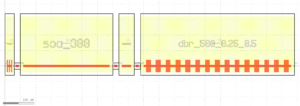To create a laser building block we will use Nazca ‘Cell’ object. Nazca calls a building block a ‘Cell’ object, because it will be exported as a cell in GDS. The new laser building block can be put several times in the mask.

```# example created by Bright Photonics

import nazca as nd
import nazca.demofab as demo

with nd.Cell(name='laser') as laser:
#create a iso cell for reuse
iso = demo.isolation_act(length=20)
#draw the laser
demo.dbr(length=30).put(0)
iso.put()
demo.soa(length=300).put()
iso.put()
demo.phase_shifter(length=50).put()
iso.put()
demo.dbr(length=500).put()

laser.put(0)
laser.put(0, 300)
laser.put(0, 600)

nd.export_gds()```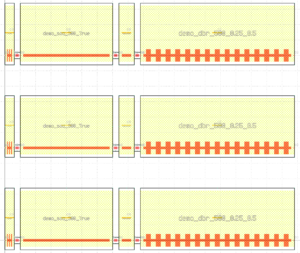We can also add Pins to the laser building block in order to connect it to the rest of the layout, for example to interconnect laser metal pads to external DC pads.

```# example created by Bright Photonics

import nazca as nd
import nazca.demofab as demo

def dbr_laser(Ldbr1=50, Ldbr2=500, Lsoa=750, Lpm=70):
"""Create a parametrized dbr laser building block."""
with nd.Cell(name='laser') as laser:
#create a iso cell for reuse
iso = demo.isolation_act(length=20)

#draw the laser
s2a = demo.s2a().put(0)
dbr1 = demo.dbr(length=Ldbr1).put()
iso.put()
soa = demo.soa(length=Lsoa).put()
iso.put()
phase = demo.phase_shifter(length=Lpm).put()
iso.put()
dbr2 = demo.dbr(length=Ldbr2).put()
a2s = demo.a2s().put()

# add pins to the laser building block
nd.Pin('a0', pin=s2a.pin['a0']).put()
nd.Pin('b0', pin=a2s.pin['b0']).put()
nd.Pin('c0', pin=dbr1.pin['c0']).put()
nd.Pin('c1', pin=soa.pin['c0']).put()
nd.Pin('c2', pin=phase.pin['c0']).put()
nd.Pin('c3', pin=dbr2.pin['c0']).put()
return laser

#place the laser block
laser1 = dbr_laser().put(0)

demo.shallow.strt(length=100).put(laser1.pin['a0'])
demo.shallow.strt(length=200).put(laser1.pin['b0'])

for i, pinname in enumerate(['c0', 'c1', 'c2', 'c3']):

nd.export_gds()```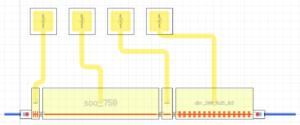## Related Tutorials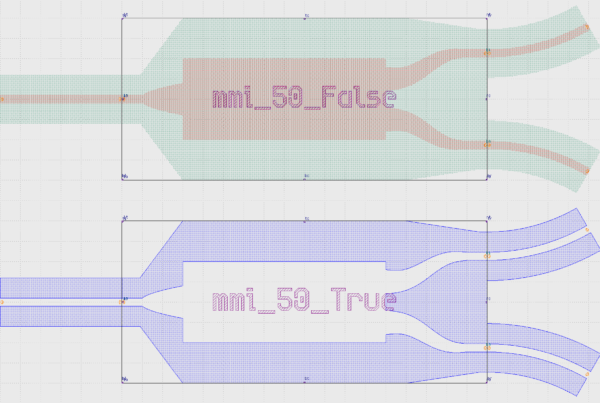In this example we show how to create an inverted MMI with custom interconnects.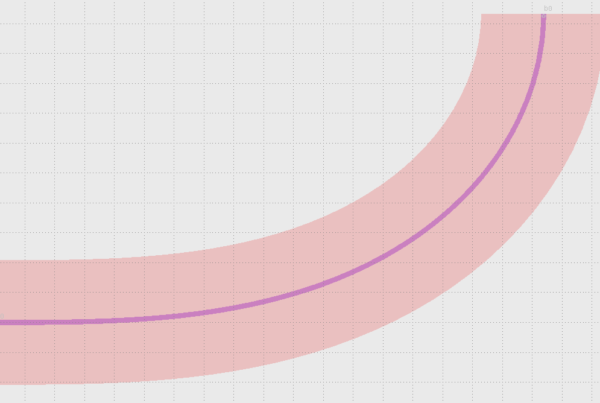In this example we show how to use Euler bends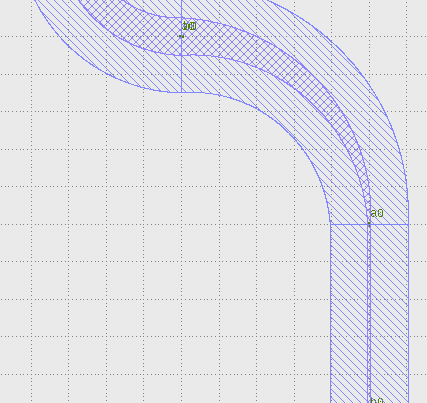In this example we show how to create a free form parametric curve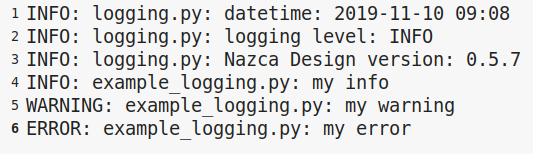In this example we show how to log your layout.